Next: 6.4 Vertical Discretization of Up: 6.3 Formulation of the Previous: 6.3.2 Resist/Substrate Interface

## 6.3.3 Vector-Matrix Notation

In Section 6.2.6 we introduced a vector-matrix notation to achieve a compact form for the ODE system. The notation is governed by the convention (6.30) combined with the index-mapping of (6.31). Using this notation the BCs (6.40) and (6.47) at z = 0 and z = h, respectively, take the form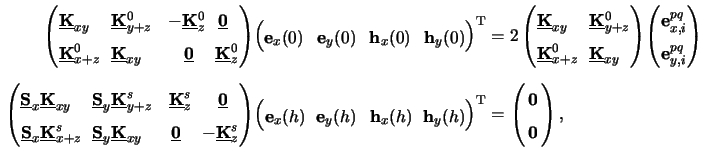whereby all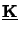-matrices as well as the two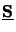-matrices are diagonal with dimension NODE x NODE. For example,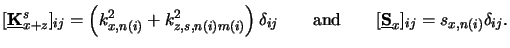(6.31)

Note that the diagonal property of the matrices reflects the mutual independence of the BCs of different harmonic frequencies (n, m).

Similarly, to the transition from (6.32) to (6.34) we comprise the individual coefficient vectors to one common vector u(z) (cf. (6.33)) and can thus further condense (6.49) to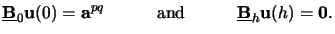(6.32)

The two boundary matrices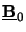and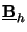are both of dimension NODE/2 x NODE, whereas the excitation vectors apq and 0 are of dimension NODE/2. At the upper interface at z = 0 a different excitation vector apq occurs for each coherent source point (p, q). The simultaneous treatment of multiple BCs will be described in Section 6.4.3 which is one of the major advantages of the proposed implementation of the differential method. Furthermore it is worth mentioning that the excitation vector at the lower interface z = h vanishes, i.e., we have the zero-vector 0, which reflects that only outgoing waves occur in the substrate due to the assumption of an infinitely extended substrate (cf. Fig. 6.1).Next: 6.4 Vertical Discretization of Up: 6.3 Formulation of the Previous: 6.3.2 Resist/Substrate Interface
Heinrich Kirchauer, Institute for Microelectronics, TU Vienna
1998-04-17